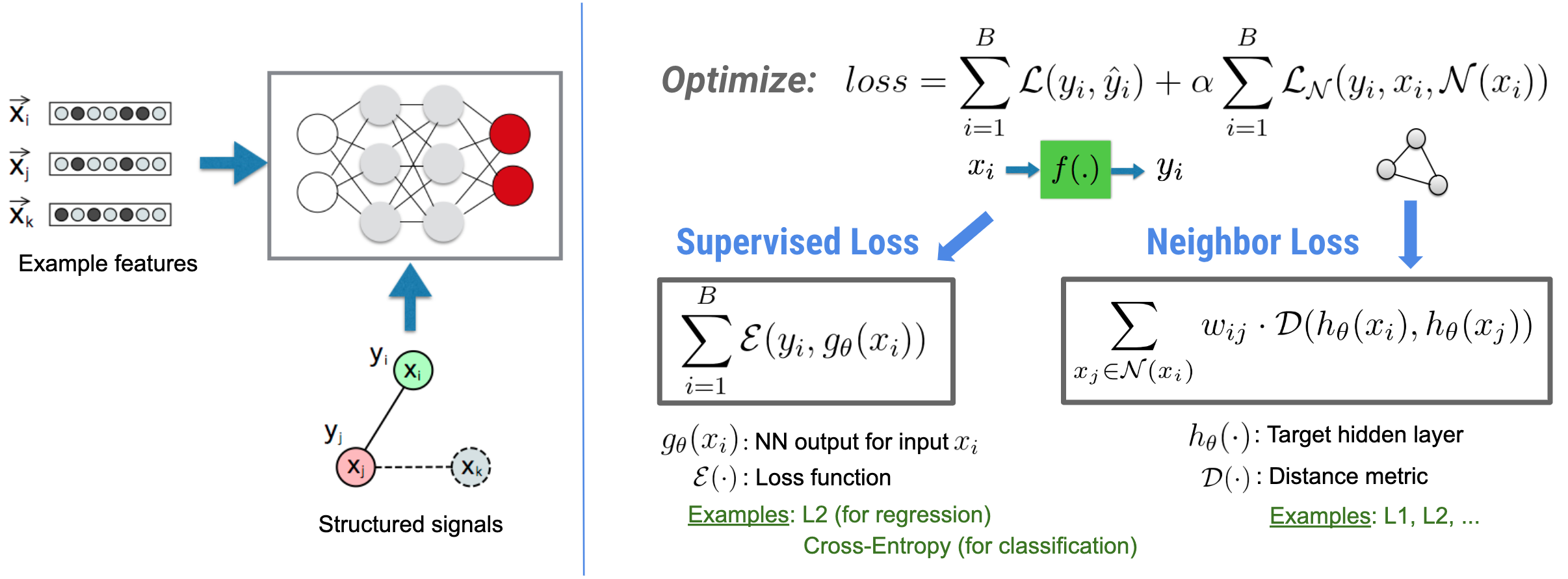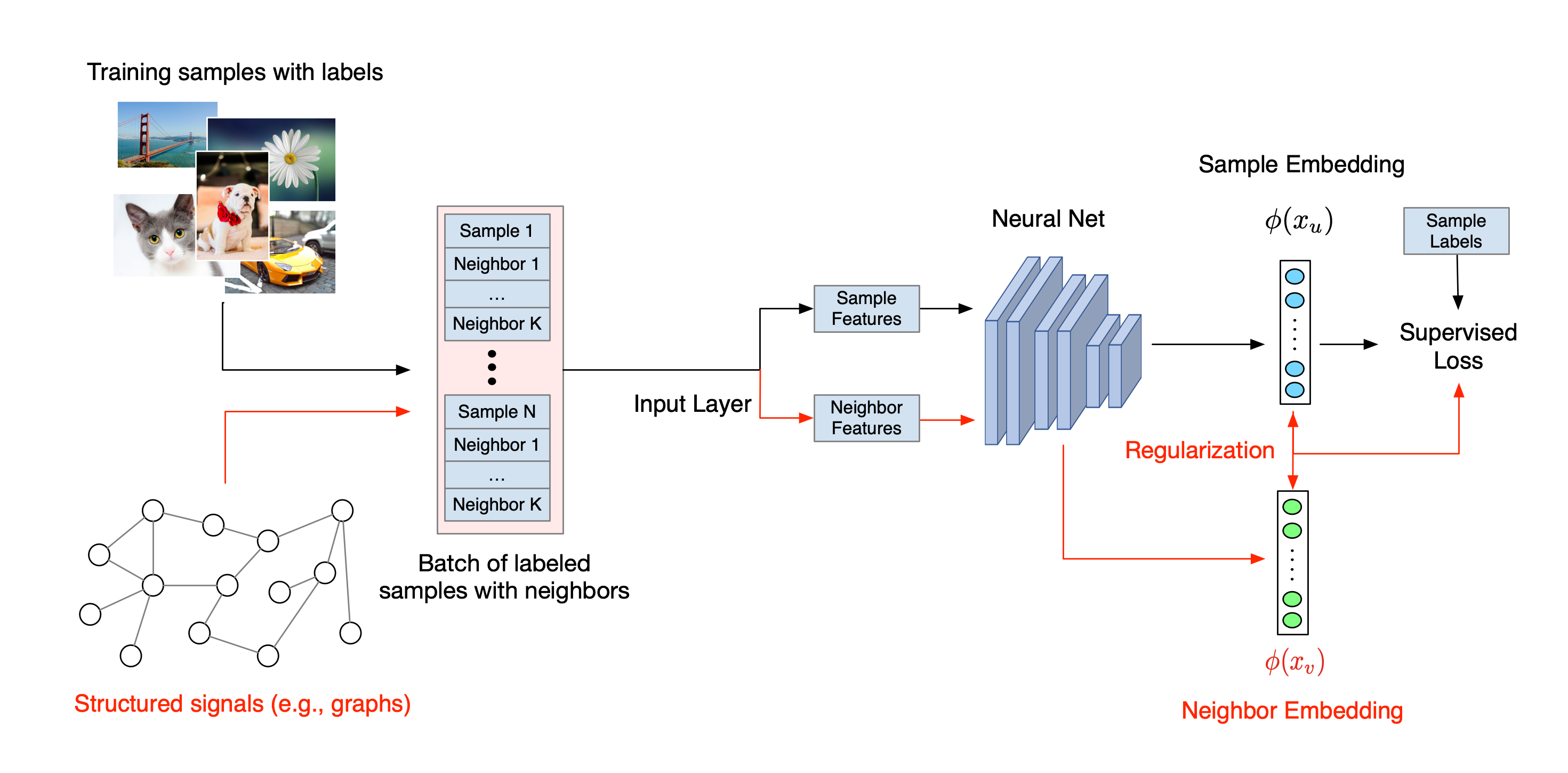Thanks for tuning in to Google I/O. View all sessions on demand

# The Neural Structured Learning Framework

Neural Structured Learning (NSL) focuses on training deep neural networks by leveraging structured signals (when available) along with feature inputs. As introduced by Bui et al. (WSDM'18), these structured signals are used to regularize the training of a neural network, forcing the model to learn accurate predictions (by minimizing supervised loss), while at the same time maintaining the input structural similarity (by minimizing the neighbor loss, see the figure below). This technique is generic and can be applied on arbitrary neural architectures (such as Feed-forward NNs, Convolutional NNs and Recurrent NNs).Note that the generalized neighbor loss equation is flexible and can have other forms besides the one illustrated above. For example, we can also select $$\sum_{x_j \in \mathcal{N}(x_i)}\mathcal{E}(y_i,g_\theta(x_j))$$ to be the neighbor loss, which calculates the distance between the ground truth $$y_i$$ and the prediction from the neighbor $$g_\theta(x_j)$$. This is commonly used in adversarial learning (Goodfellow et al., ICLR'15). Therefore, NSL generalizes to Neural Graph Learning if neighbors are explicitly represented by a graph, and to Adversarial Learning if neighbors are implicitly induced by adversarial perturbation.

The overall workflow for Neural Structured Learning is illustrated below. Black arrows represent the conventional training workflow and red arrows represent the new workflow as introduced by NSL to leverage structured signals. First, the training samples are augmented to include structured signals. When structured signals are not explicitly provided, they can be either constructed or induced (the latter applies to adversarial learning). Next, the augmented training samples (including both original samples and their corresponding neighbors) are fed to the neural network for calculating their embeddings. The distance between a sample's embedding and its neighbor's embedding is calculated and used as the neighbor loss, which is treated as a regularization term and added to the final loss. For explicit neighbor-based regularization, we typically compute the neighbor loss as the distance between the sample's embedding and the neighbor's embedding. However, any layer of the neural network may be used to compute the neighbor loss. On the other hand, for induced neighbor-based regularization (adversarial), we compute the neighbor loss as the distance between the output prediction of the induced adversarial neighbor and the ground truth label.